# Motion and Measurement of distances Class 6 Notes

## MEASUREMENT IN DAILY LIFE

• Measurement is a method that enables us to identify the quantity of anything.
• In measurement, we compare the unknown quantity of an object to the known fixed quantity of an object of the same kind, which leads us to measure the quantity of the unknown object.
• The known fixed quantity in a measurement is called a unit. For example, the length of cloth is 10 metres, so the unit of measurement here is a metre.
• The quantity equals the product of numerical value and the unit. It is written mathematically as:-
• Quantity= Numerical Value x Unit

## Physical Quantities

• The quantities that can be measured are called physical quantities. For measuring the physical quantities, we require magnitude and a fixed unit. For example, If the distance from London to Reading is 275 km, so the magnitude is 275 and its unit is km here.
• The specific physical quantities that cannot be expressed in any other quantity are called fundamental physical quantities. Some fundamental physical quantities are mass, length, time and temperature.
•
In ancient times, people use their hands, arms and feet to measure the quantities. These methods still use in the present time. The units that we measure through these methods are yards, cubit and handspan.
Yard: The distance between the endpoint of an outstretched arm to the tip of the nose.
Cubit: The distance between the elbow to the tip of the middle finger.
Foot: Measurement of the quantity by a barefoot of an adult person.
Handspan: The measurement of a quantity by the tip of the thumb to the tip of the little finger when the palm is outstretched.
These methods are not reliable because different people have different body shapes and sizes. However, if these methods apply individually then they can be effective. For example, tailors use the handspan method to measure the arm of the customers to make the sleeves.

Standard Units of Measurement
• The standard units of measurement introduced to measure accurate quantity of any object.
• In 1960, the General Council of Weights and Measures organised where all the scientists gathered to decide the uniform system of units acceptable all over the world.
• The standard system unit is called the SI system(‘Systeme International d’Unites’ in French). Table 1 shows the SI units of fundamental quantities.

 Fundamental quantities SI units Unit Symbol Length metre m Mass Kilogram Kg Time second s

Table 1: SI units of fundamental quantities.

A few other SI systems are:-
• CGS (Centimetre for length, Gram for mass and Second for time),
• MKS (Metre for length, Kilogram for mass and Second for time),
• FPS (Foot for length, Pound for mass and Second for time).
• The measurement of smaller quantities is called submultiples of units. They represent with the factor of 1/10, 1/100 and 1/1000, etc.
• The measurement of larger quantities is called multiples of units. They represent with the factor 10, 100 and 1000, etc.
• Both multiples and submultiples have prefixes and symbol.
Table 2 shows the prefixes of multiples and submultiples.

 Prefixes Symbol Submultiples/Multiples deci d Submultiple: 1/10 centi c Submultiple: 1/100 milli m Submultiple: 1/1000 Kilo k Multiple: 1000 Hecto h Multiple: 100 Mega M Multiple: 10000

Table 2: Prefixes used in SI units.

## CORRECT MEASUREMENT OF LENGTH

We use suitable instruments to measure the quantity of an object.
Measuring Length
• The measurement of length concerns the length, width, thickness, height and distance of any object.
• There are several instruments used to measure the length. For example, a ruler, metre rod and a measuring tape, etc.
• A ruler has units of centimetre and millimetre. It is used to measure smaller quantities(Figure 2).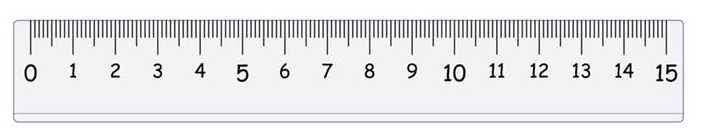Figure 2: A ruler.
A metre rod is used by tailors to measure the length of the cloth. The unit of metre rod is fixed to one metre(Figure 3).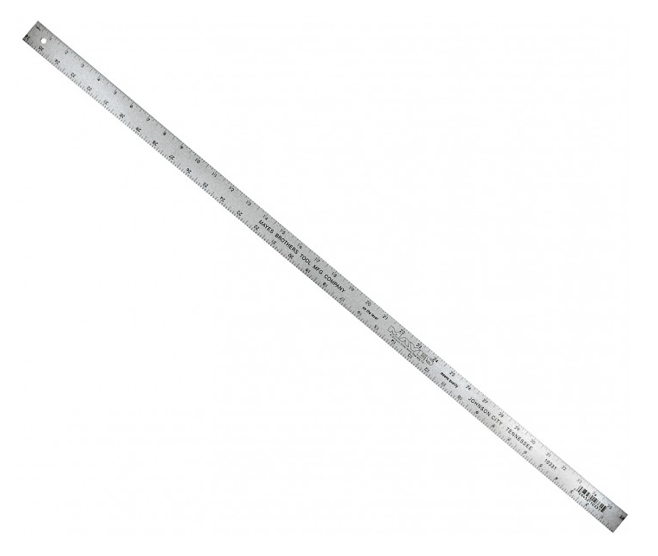Figure 3: A metre rod.

Correct way to use a ruler/scale
The following steps we need to follow while measuring anything by a ruler/scale:-
• Place the scale exactly at the tip of the object.
• Do not observe the object at different angles while measuring because this causes parallax error(Figure 4).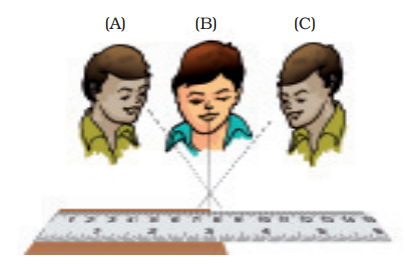Figure 4: Parallax error: Observation at different angles.

## Measuring Length of a Curved Line

We cannot measure a curved line by a ruler or metre rod. Therefore, we follow certain methods to measure the curved line:-
i. Take a long thread and mark one of its ends.
ii. Put the marked end of the thread at the starting of the curved line by using your thumb.
iii. Put the other end of the thread to the other side of the curved line by another thumb of your other hand.
iv. Lastly, stretch the thread to measure the the length(Figure 5).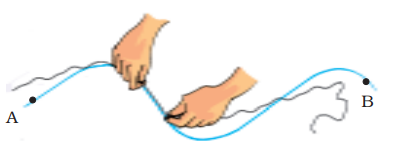Figure 5: Measuring a length of curved line by thread.

The divider method:
i. Take a pair of dividers and separate them with the help of a scale.
ii. Put the one tip of the divider at the one end of the curved line and another tip of divider at the curved line by taking markings.
iii. Repeat this process until the whole line is divided into segments(Figure 6).
iv. If any segment is left over then measure the length by a formula:-
The length of a curved line= (Number of segments of equal lengths x distance between the tips of the divider) + length of the left over part.

## ESTIMATION OF MEASUREMENT

The ability of guessing the value of anything without calculating the accurate value of the object is called the estimation. For example, by planning a trip, we assume the approximate cost and time.

## MOTION

• The changing in the position of any object concerning time regarding the effects of its surroundings is called a motion. For example, driving a car.
• If an object is fixed at a particular position then there is no changing of the position called stationary or at the rest. For example, trees are fixed at a particular place.
• Living things move on their own, but non-living things do not move on their own. For example, a car needs a driver and fuel to move.

## Rest and Motion are Relative

The state of being at rest or in motion is always relative to a certain object. For example, suppose you and your father sitting in a car and your mother is standing in the street. When the car moves, your father is at rest to you but not to your mother.

### TYPES OF MOTION

The motion is divided into several categories:-
Translatory Motion
When all parts of an object in motion moving through an equal distance in the same direction and time are called translatory motion. For example, a car moving in a straight path covers an equal distance in the same direction and time(Figure 7 a). Translatory motion can be linear or curved. The linear translatory motion is called rectilinear motion, whereas the curved translatory motion is called curvilinear motion. The best example of curvilinear motion is throwing a basketball in the basket(Figure 7 b).
Rectilinear motion: The motion of any object takes place in a straight line with respect to the time and in the same direction.Curvilinear motion: The motion of any object takes place along a curved path with respect to the time and in the same direction.

Circulatory motion
The motion of any object takes place in a circular path is called a circulatory motion. For example, (Figure 8).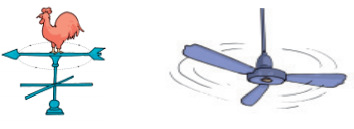Figure 8: Circulatory motion.

Rotational motion
The motion of any object takes place around an axis passing through its centre is called a rotational motion. For example, the wheels of a bicycle, a merry-go-round, blades of a fan (Figure 8).

Oscillatory motion
When a body repeatedly changes its position in a to and fro motion about its mean position is called an oscillatory motion. One complete cycle from the initial point to the extreme point is called one oscillation. For example, a swing hammock and a pendulum(Figure 9).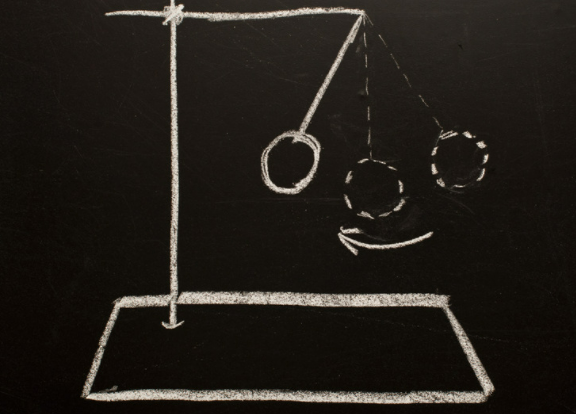Figure 9: Oscillatory motion.

Vibratory motion
When a body moves vibrates about its mean position is called a vibratory motion. For example, the strings of a guitar, the skin of the drum and the needle of a sewing machine, etc(Figure 10).Figure 10: Examples of vibratory motion.

Periodic Motion
When a body moves in a fixed interval of time then such motion is called periodic motion. The best example of a periodic motion is a clock and other examples are mentioned above(Figure 11).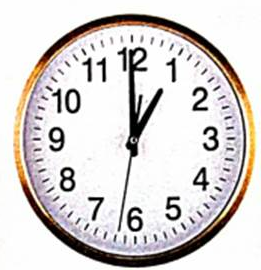Figure 11: Periodic motion.

Non-periodic motion
When a body changes its position without concerning the fixed interval of time then it is called non-periodic motion. For example, the movement of clouds in the sky, a bicycle moving in a straight line and driving a car.### Practice Question

Question 1 What is $\frac {1}{2} + \frac {3}{4}$ ?
A)$\frac {5}{4}$
B)$\frac {1}{4}$
C)$1$
D)$\frac {4}{5}$
Question 2 Pinhole camera produces an ?
A)An erect and small image
B)an Inverted and small image
C)An inverted and enlarged image
D)None of the above